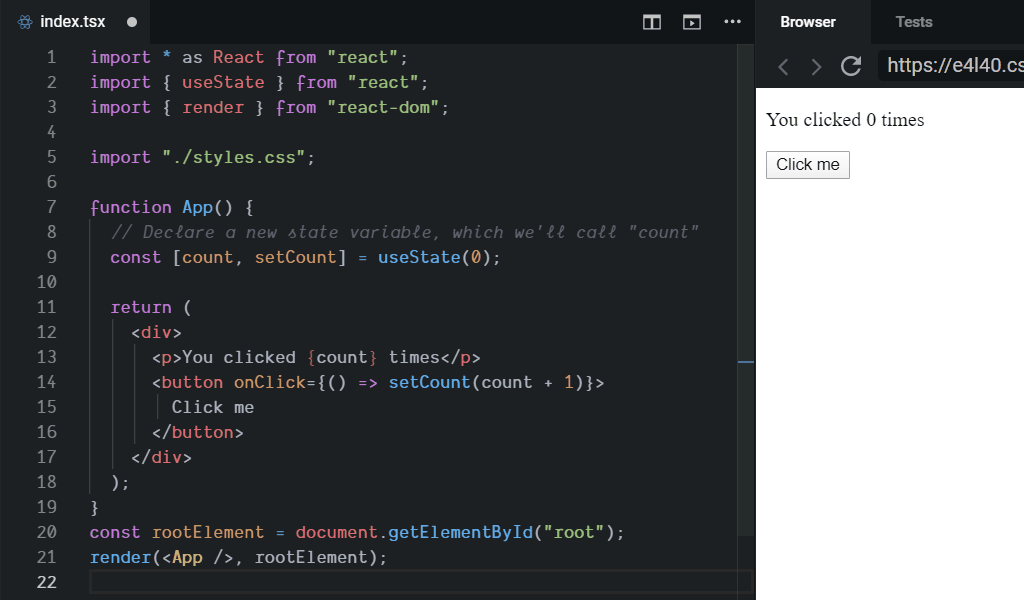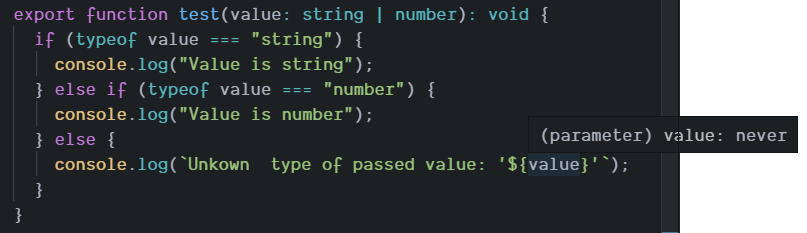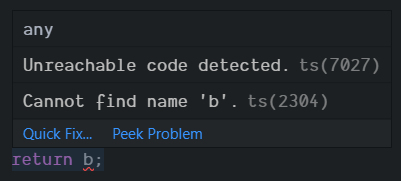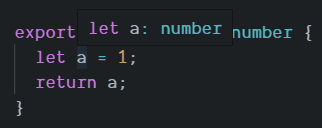Why use TypeScript?

I don't want to give you an official definition. Instead of it, I wish to share my definition:

TypeScript is the way to develop a low bug code that is easy to maintain and share among people within a long time.

Here is the list of what TypeScript won't do for you:

• Write a bug-free code.
• Avoid the run-time errors.
• Enhance debugging.
• Guarantee that the returned data at the run-time will be of the expected type.
• Make the code more readable.
• Simplify the integration of the third-party frameworks/libraries/code.
• Make the code self-documented.

So, why use TypeScript anyway? (only the top of the list):

• You will spend less time to understand a new codebase.
• You will know what type of data is expected and/or returned by any part of the code.
• You will know the structure of the data you're working with.
• You will have the ability to lint your code in real-time.
• Any changes will affect all the parts of code, so you will be forced by the linter/compiler to update all of the connected code.
• It will be much easier to collaborate on the development.
• Others can benefit from your code with ease.

I hope that now you can judge by yourself if TypeScript worth your time and effort.

A short overview of the abilities of TypeScript: built-in types, user-defined types, interfaces, generics, iterators and generators, symbols, type inference, etc.

Below goes a short overview of the listed features.

Built-in types

The basic types are reflecting types of JavaScript with some extended types: boolean, number, string, array, tuple, enum, any, void, null and undefined, never, object.

Tuple

Here is the original definition:

Tuple types allow you to express an array with a fixed number of elements whose types are known, but need not be the same.

So, this means that you can describe an array with fixed length and exact types. Here's an example:

``````// Declare a tuple type
let x: [string, number];
// Initialize it
x = ["hello", 10]; // OK
// Initialize it incorrectly
x = [10, "hello"]; // Error
``````

Thus, every time you use hooks in React you are using a tuple!

``````// Declare a new state variable, which we'll call "count"
const [count, setCount] = useState(0);
``````Enum

Enum is a way to give more friendly names to sets of numeric values.

They are simplifying work with numeric values. Instead of memorizing the set of numbers you can define an enum like this:

``````enum Color {Red = 1, Green = 2, Blue = 4}
let c: Color = Color.Green;
``````
Any

If for some reason, it is impossible to define the type of data, you can use a special type named any.

This type can be used during the early stages of an application outlining. Or if the content comes from some external source, e.g. third-party library.

``````let notSure: any = 4;
notSure = "maybe a string instead";
notSure = false; // okay, definitely a boolean
``````

Please, avoid using this type in production releases because it makes meaningless to use TypeScript at all.

Void

This is the moment when I thought: "JavaScript already has null, undefined, NaN, Infinity. TypeScript adds type named any. What is void?".

The answer is simple: the void type is used for functions that won't return anything. Like this:

``````function log(value: string): void {
console.log(value)
}
``````

Of course, it is possible to declare a variable with that type. But it is useless. The only value that can be assigned in this case is null.

Never

As with the previous type, I was totally confused when I've found yet another type!

But once again, everything is simple and logical in this case.

The type never is used to indicate something that will never happen.

For example:

``````// Function returning never must have unreachable end point
function error(message: string): never {
throw new Error(message);
}
``````

The function above will interrupt the code execution that is why it's return type is never.

Another situation is when the edge case is impossible. Take a look at the screenshot below:In the first and second cases, the parameter value will be treated as a string and as a number respectively.

The last condition is a good place to throw an error.

Variable declarations

There are two main differences between variable declarations in JavaScript and TypeScript:

• TypeScript by default uses let and const instead of var.
• Every declaration without assignment must have a type definition.

Why? Because it is safer.

let and const vs var

TypeScript introduces slightly different ways to declare a variable. Let's have a look at them!

let

The let keyword was introduced to JavaScript in ES2015. The main difference versus var is that it is block scoped. Let's have a look at two examples to catch the difference.

``````for (var i = 0; i < 3; i++) {
setTimeout(function() { console.log(i); }, 1)
}
``````

The output of the above script will be this:

``````3
3
3
``````

Ok, now let's replace var with let:

``````for (let i = 0; i < 3; i++) {
setTimeout(function() { console.log(i); }, 1)
}
``````

In the latter case the output will look like this:

``````0
1
2
``````

When you use the let declaration the variable is bound to a scope of execution. So, in each iteration, you will have a new instance of the variable with the current value, which will be passed to a setTimeout call.

When you use the var declaration you have a single variable for all of the iterations. So, the final value will be stored in a single variable that will be later passed to function within the setTimeout call.

One more cool feature is that the variable can't be used before the declaration in the case of let.

The below example works perfectly in case of a var declaration.

``````a += 1;

var a = 1;
``````

Because let declaration is bound to the scope, you are unable to return the value defined within the nested scope. The below example will raise an exception:

``````export function f(): number {
let a = 1;

if (true) {
let b = a + 1;
}

return b;
}
``````Yet another cool feature is that the let declaration won't let you make a shadow-declaration.

``````export function f(): void {
let x = 1; // OK
let x = 2; // this line will raise an error
}
``````
const

The const keyword allows you to declare variables in a different way. These declarations are working like let with one significant difference: their value cannot be changed.

But wait, there is one very important fact: you still can alter values within objects and arrays!

Let's have a look at examples.

``````const a = true;
a = false; // this line will raise an error
``````

That was a simple type. Now let's have a look at the array type:

``````const s = [];

s = [1, 2, 3]; // this line will raise an error

s.push(1); // OK

const q = s.pop(); // OK
``````

The same is true for objects:

``````const w = {
a: 1,
b: 2
};

w = {}; // this line will raise an error

w.c = 3; // error

w.a++; // OK
``````
Type definition

In case if a variable is declared through the assignment of value/function call with a known type, there is no need to define type explicitly.But if you don't know the initial value you should make a declaration like this:

``````export class User {
private name: string;
private age: number;

constructor(name: string, age: number) {
this.name = name;
this.age = age;
}

getName(): string {
return this.name;
}

getAge(): number {
return this.age;
}
}
``````

Did you notice that the constructor declaration does not have a return type? It is one of the special cases when the return type cannot be defined.

Let's examine this example!

Firstly, take a look at how fields name and age are declared:

``````class User {
private name: string;
private age: number;
}
``````

We have not only defined the fields but also have declared their types. Now, TypeScript will not allow assigning a value of the wrong type to these properties of a class.

``````class User {
private name: string;

constructor(value: number) {
this.name = value; // this line will raise an error
}
}
``````

Next, let's have a look at how methods of the class are defined:

``````export class User {
private age: number;

constructor(age: number) {
this.age = age;
}

getAge(): number {
return this.age;
}
}
``````

The getAge function has a return type defined right after function contract declaration:

``````getAge(): number {
return this.age;
}
``````

This declaration disallows us to return any type except defined:

``````getAge(): number {
if (typeof this.age !== 'number') {
return 'wrong value!'; // this line will raise an error
}

return this.age;
}
``````
Interfaces

Interfaces are a way to describe the structure of an object.

Now, let's take a look at the example:

``````interface IUser {
name: string;
age?: number;
}
``````

It is a good practice to start the names of interfaces with I. Thus it will be easier to distinguish interfaces from other types in the declaration clauses.

You are already familiar with the first type of declaration:

``````interface IUser {
name: string;
}
``````

The above definition requires that the object must have field name of type string.

The next definition instructs typescript to require that the object has a read-only field approved of type boolean:

``````interface IUser {
}
``````

The last definition introduces the declaration of an optional field:

``````interface IUser {
age?: number;
}
``````

So, the field age of type number is optional in this interface.

Here is an example of usage:

``````interface IUser {
name: string;
age?: number;
}

const user1: IUser = {}; // error
const user2: IUser = { name: 'John' }; // error
const user2: IUser = { name: 'John', approved: true }; // OK

user2.name = 'Bob'; // OK
user2.approved = false; // this line will raise an error
user2.age = 20; // OK
``````

TypeScript will force you to follow the interface definition. Thus you and others who will use your code will avoid making mistakes. Ain't it superb?

Functions

As you have seen above, definitions of functions can also be improved by TypeScript.

Here is an example:

``````function double(value: number, roundup?: boolean): number {
if (roundup === true) {
value = Math.round(value);
}

return value * 2;
}

double(10.5); // result 21
double(10.5, true); // result 22
``````

This function tells us that it expects one required parameter of type number and another optional parameter of type boolean. Also, the declaration tells us that this function will return a number.

Generics

Generics are used in case if a type can be replaced or inferred from usage.

The next examples are very simplified to show the gist.

Let's say that you need to perform the same operation for different types of data:

``````function fNumber(arg: number): number {
return arg;
}

function fString(arg: string): string {
return arg;
}

function fBoolean(arg: boolean): boolean {
return arg;
}

fNumber(true); // error
fString(1); // error
fBoolean('string'); // error
``````

Instead of duplicating code over and over again, we can use a generic function!

``````function f<T>(arg: T): T {
return arg;
}

f(true); // OK
f(1); // OK
f('string'); // OK
``````

In the latter example, the type is inferred from the argument. So, we can use even complex types!

``````interface IData {
valid: boolean;
}

function f<T>(arg: T): T {
return arg;
}

const data: IData = { valid: true };

f(data); // OK
``````
Types overview

To catch the difference between types and interfaces, please, refer to this post:

Here I will give you a glimpse of how types can help you. Let's take a look at an example:

``````type ReturnTypeFunction = () => boolean;
type ReturnType = boolean | ReturnTypeFunction;

interface IButton {
disabled: ReturnType;
}
``````

Please, note that type names should follow the CamelCase convention.

Now let's examine the example.

Firstly, we declaring type which refers to a function which returns a boolean value:

``````type ReturnTypeFunction = () => boolean;
``````

Next, we define a union type which combines raw boolean value with the alias type which we declared above:

``````type ReturnType = boolean | ReturnTypeFunction;
``````

Lastly, we use our union type as a type for a field of the interface:

``````interface IButton {
disabled: ReturnType;
}
``````

Later on, you can extend this union type with generic to avoid code duplication:

``````type ReturnTypeFunction<T> = () => T;
type ReturnType<T> = T | ReturnTypeFunction<T>;

interface IButton {
disabled: ReturnType<boolean>;
}
``````
Conclusion

I hope that you will find this brief overview helpful.

Summary:

TypeScript will help to effectively build your application.

Other people (including the future you) will benefit from statically defined types.

Every change will affect all parts of an application.

You will know the structure of the data.

Real-time linters will help you to follow the defined types.

PS:

If you have found any errors or if you wish to clarify something, please, let me know!

Thank you!

# Update

If you want to know how the cover image was generated, check out this post:

This post is also available on DEV.